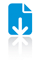Best-Order Streaming model

ContentAbstract

We study a new model of computation, called best-order stream, for graph problems. Roughly, it is a proof system where a space-limited verifier has to verify a proof sequentially (i.e., it reads the proof as a stream). Moreover, the proof itself is just a specific ordering of the input data. This model is closely related to many models of computation in other areas such as data streams, communication complexity, and proof checking, and could be used in applications such as cloud computing. In this paper we focus on graph problems where the input is a sequence of edges. We show that even under this model, checking some basic graph properties deterministically requires linear space in the number of nodes. We also show that, in contrast with this, randomized verifiers are powerful enough to check many graph properties in polylogarithmic space.TopTopTopTop